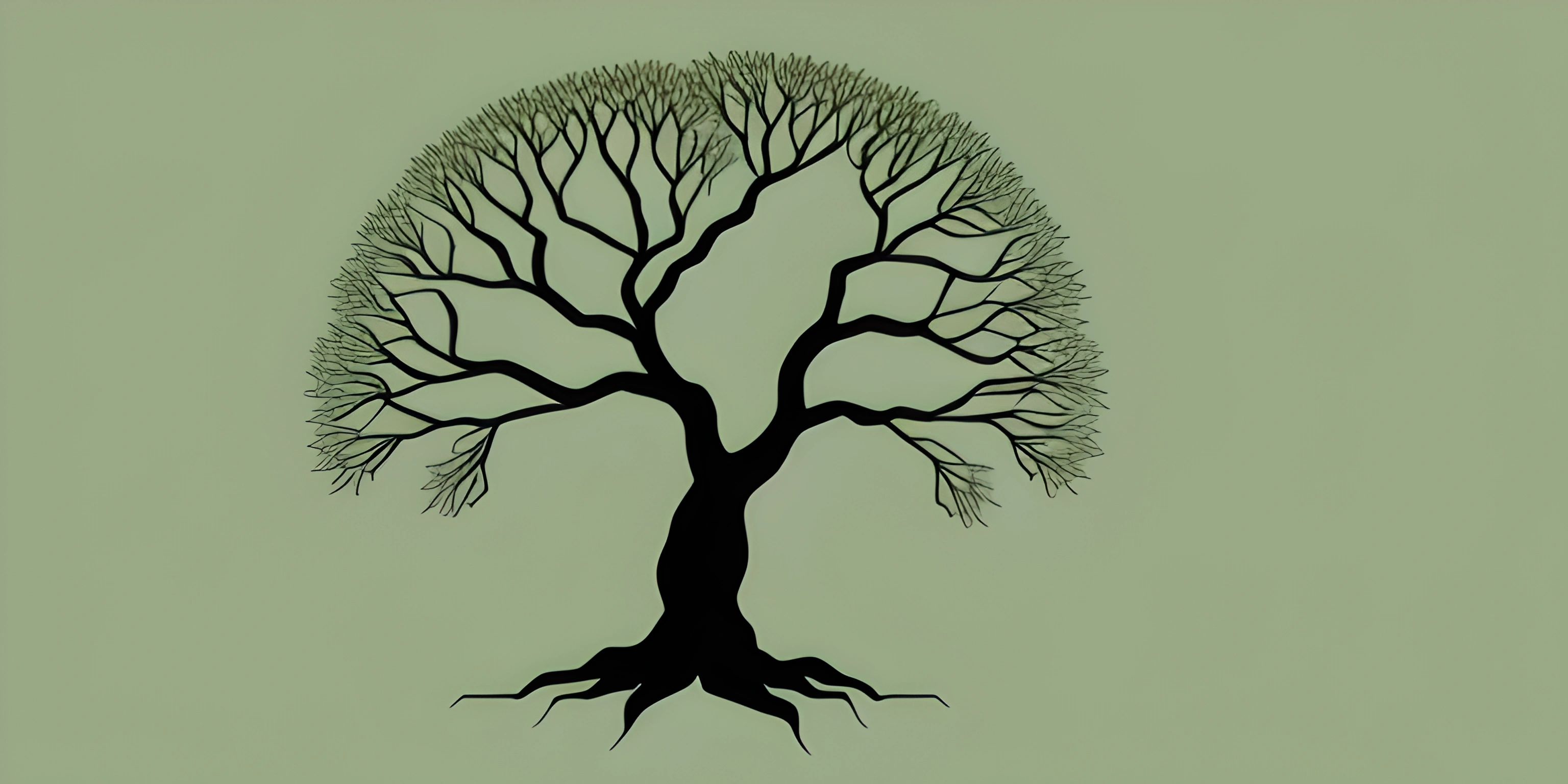# Introduction to TreesNote: this page has been created with the use of AI. Please take caution, and note that the content of this page does not necessarily reflect the opinion of Cratecode.

Trees are one of the most important data structures in computer science. They are everywhere, from file systems to language parsers, and from search engines to databases. If you've ever wanted to understand how trees work, you've come to the right place. Let's dive into the world of trees!

## What is a Tree?

A tree is a non-linear, hierarchical data structure consisting of nodes connected by edges. It starts with a single node, called the root, and branches out to other nodes, forming a tree-like structure. Each node in a tree can have zero or more children, and every child node has exactly one parent, except for the root, which has no parent.

Here's a simple example of a tree:

``````          A
/   \
B     C
/ \   / \
D   E F   G``````

In this tree, `A` is the root, and it has two children, `B` and `C`. The nodes `D`, `E`, `F`, and `G` are all leaf nodes, meaning they have no children.

## Basic Terminology

Now that you have a basic understanding of what a tree looks like, let's go over some common tree-related terms.

• Node: A unit in a tree that contains data and links to its children.
• Edge: A connection between two nodes.
• Root: The top-most node in a tree, which has no parent.
• Parent: A node that has one or more child nodes.
• Child: A node that is directly connected to another node (its parent).
• Sibling: Nodes that share the same parent.
• Leaf Node: A node with no children.
• Level: The distance of a node from the root, with the root being at level 0.
• Height: The length of the longest path from the root to a leaf node.

## Types of Trees

There are several types of trees you'll encounter in computer science:

• Binary Tree: A tree in which each node has at most two children. This is the most common type of tree you'll encounter.
• Binary Search Tree: A binary tree with the additional property that the value of every node in its left subtree is less than or equal to the node's value, and the value of every node in its right subtree is greater than or equal to the node's value.
• AVL Tree: A self-balancing binary search tree that maintains a height-balanced property, ensuring that the tree remains efficient for operations.
• Trie: A tree-like data structure used for efficiently storing and retrieving strings or sequences of characters.

This is just the beginning of your journey into the world of trees. As you explore further, you'll learn about various tree operations, algorithms, and tree traversal techniques. Get ready to branch out and expand your knowledge of trees!

## FAQ

### What is a tree data structure?

A tree data structure is a hierarchical structure that consists of nodes connected by edges. Each node in a tree has a parent (except the root node) and zero or more children. Trees are often used to represent relationships and hierarchies in real-world scenarios, such as representing an organization's structure or a file system.

### What is the root node in a tree?

The root node is the topmost node in a tree data structure that has no parent. All other nodes in the tree can be reached by following edges from the root node, which serves as the starting point for traversals and operations on the tree.

### Can you explain the terms parent, child, and sibling in the context of a tree?

In a tree data structure, the terms parent, child, and sibling are used to describe the relationships between nodes:

• Parent: A node that has one or more child nodes connected to it.
• Child: A node that is connected to a parent node.
• Sibling: Nodes that have the same parent node. They are essentially "neighbors" in the tree structure.

### How do you calculate the height and depth of a tree?

The height and depth of a tree are measures of its size and structure:

• Height: The height of a tree is the length of the longest path from the root node to a leaf node. A leaf node is a node with no children. The height of an empty tree is usually considered to be -1 or 0, depending on the context.
• Depth: The depth of a node is the length of the path from the root node to that particular node. The depth of the root node is always 0, as it's the starting point.

### What are some common types of trees used in computer science?

Several types of trees are commonly used in computer science, including:

• Binary Trees: Each node has at most two children (left and right).
• Binary Search Trees: A binary tree where the value of each node is greater than or equal to the values of all nodes in its left subtree and less than or equal to the values of all nodes in its right subtree.
• AVL Trees: A self-balancing binary search tree that ensures the height difference between the left and right subtrees of any node is at most 1.
• Trie (Prefix Trees): A tree-like data structure used to store a dynamic set or associative array, where the keys are usually strings.
• B-Trees: A balanced tree data structure commonly used in databases and file systems to enable efficient search, insertion, and deletion operations.